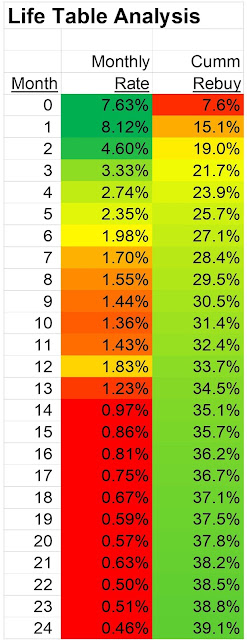## August 13, 2009

### OMS: Up-Selling and Cross-Selling

Up-Selling and Cross-Selling merchandise to a customer is an established e-commerce best practice, right? Retailers love to capture the additional margin dollars at the end of a transaction by offering the customer the opportunity to add an item to her order.

With a standard Web Analytics software tool, we can identify how customers respond to a cross-sell or up-sell opportunity.

With the Online Marketing Simulation (OMS), we can see what the downstream impact is of a customer who responds to an up-sell or cross-sell opportunity.

Let's simulate 1,000 new customers who purchased three items for \$50 each via paid search. In my dataset, I have eight merchandise divisions. Let's assume that the customer purchased three items from merchandise division #3. Here's a five year simulated run for this customer:
• 12 Month Repurchase Rate = 24%.
• Future Demand: Year 1 = \$51,000, Year 2 = \$35,000, Year 3 = \$28,000, Year 4 = \$25,000, Year 5 = \$23,000.
• Merchandise Division #1 Buyers: Year 1 = 22, Year 2 = 15, Year 3 = 12, Year 4 = 11, Year 5 = 11.
• Merchandise Division #2 Buyers: Year 1 = 26, Year 2 = 18, Year 3 = 15, Year 4 = 13, Year 5 = 12.
• Merchandise Division #3 Buyers: Year 1 = 128, Year 2 = 72, Year 3 = 52, Year 4 = 45, Year 5 = 42.
• Merchandise Division #4 Buyers: Year 1 = 113, Year 2 = 65, Year 3 = 48, Year 4 = 41, Year 5 = 38.
• Merchandise Division #5 Buyers: Year 1 = 66, Year 2 = 42, Year 3 = 34, Year 4 = 30, Year 5 = 28.
• Merchandise Division #6 Buyers: Year 1 = 20, Year 2 = 15, Year 3 = 13, Year 4 = 12, Year 5 = 11.
• Merchandise Division #7 Buyers: Year 1 = 28, Year 2 = 19, Year 3 = 15, Year 4 = 13, Year 5 = 13.
• Merchandise Division #8 Buyers: Year 1 = 36, Year 2 = 25, Year 3 = 19, Year 4 = 17, Year 5 = 16.

Ok, those metrics don't have much meaning unless you have something to compare them to. Now let's assume that you, the online marketer, were able to cross-sell or up-sell this customer one additional item, a \$50 item in Merchandise Division #7.

How does this one additional item, a \$50 item in Merchandise Division #7, impact the future trajectory of this customer? We'll pop the results into the OMS ... let's see what the simulation tells us!

• 12 Month Repurchase Rate = 41%.
• Future Demand: Year 1 = \$104,000, Year 2 = \$68,000, Year 3 = \$48,000, Year 4 = \$38,000, Year 5 = \$33,000.

Let's just stop right there. The simulation suggests that a new paid search customer with this one additional item from a different merchandise division is instantly worth between 50% and 100% more. Clearly, your mileage will vary, some of you will experience no incremental long-term value, some of you will experience double or triple this outcome. The goal, of course, is for you to strategically think whether this issue has applicability to your business.

Here's how customers purchased from the eight merchandise divisions in my dataset.

• Merchandise Division #1 Buyers: Year 1 = 37, Year 2 = 28, Year 3 = 21, Year 4 = 18, Year 5 = 16.
• Merchandise Division #2 Buyers: Year 1 = 39, Year 2 = 33, Year 3 = 25, Year 4 = 21, Year 5 = 19.
• Merchandise Division #3 Buyers: Year 1 = 227, Year 2 = 123, Year 3 = 79, Year 4 = 61, Year 5 = 53.
• Merchandise Division #4 Buyers: Year 1 = 225, Year 2 = 119, Year 3 = 78, Year 4 = 61, Year 5 = 52.
• Merchandise Division #5 Buyers: Year 1 = 86, Year 2 = 73, Year 3 = 55, Year 4 = 45, Year 5 = 40.
• Merchandise Division #6 Buyers: Year 1 = 36, Year 2 = 28, Year 3 = 22, Year 4 = 19, Year 5 = 17.
• Merchandise Division #7 Buyers: Year 1 = 44, Year 2 = 35, Year 3 = 25, Year 4 = 21, Year 5 = 18.
• Merchandise Division #8 Buyers: Year 1 = 55, Year 2 = 43, Year 3 = 32, Year 4 = 26, Year 5 = 23.

Pay close attention to Merchandise Division #7. This was the division where the up-sell / cross-sell item was purchased from. There is some improvement in the number of buyers in this division. Now pay attention to Merchandise Divisions #3 and #4 --- these divisions are expected to get a significant bump in customers.

Yes folks, your actions in one area of your business cause unexpected changes in the business performance of another area of your business. In this example, the cross-sell of one item from Merchandise Division #7 causes Merchandise Division #4 to experience a significant improvement in performance within this customer segment (in fact, almost all divisions experience improvement). Merchandise Division #4, of course, had no role in either simulated transaction --- it simply benefits from something that happens elsewhere in your business.

This is what Advanced Web Analytics, specifically, the Online Marketing Simulation (OMS) is all about. We're looking to see how the decisions we make today impact the future of our business. Most Web Analytics applications look backward, measuring what happened in the past. The OMS environment looks forward.